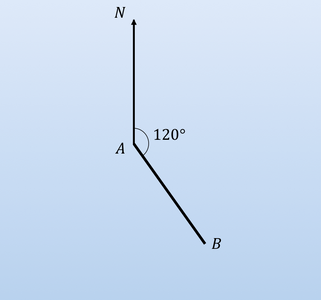# Bearings and Scale Factors - Mathematics

• Created by: v.a..
• Created on: 18-04-19 14:48

## Bearings• A bearing is a measure of direction which is measured in degrees clockwise from north.
• To measure the bearing from A to B, draw a vertical line up from A and label it as North.
• Then using a protractor measure the angle clockwise from this vertical line to the line between A and B.
• Bearings are always given as 3 digits:
• 120°, 030°, 009°.
1 of 2

## Scale Factor and Enlargement

When a shape is enlarged, its lengths are all multiplied by S.• for length• for area - When a 2D shape is enlarged by a scale factor S, because all the lengths are multiplied by S, its area will be multiplied by S².• for volume - When a 3D shape is enlarged by a scale factor S all the lengths are multiplied by S.
• The areas in the shape will be multiplied by S² and the volumes will be multiplied by S³.
2 of 2